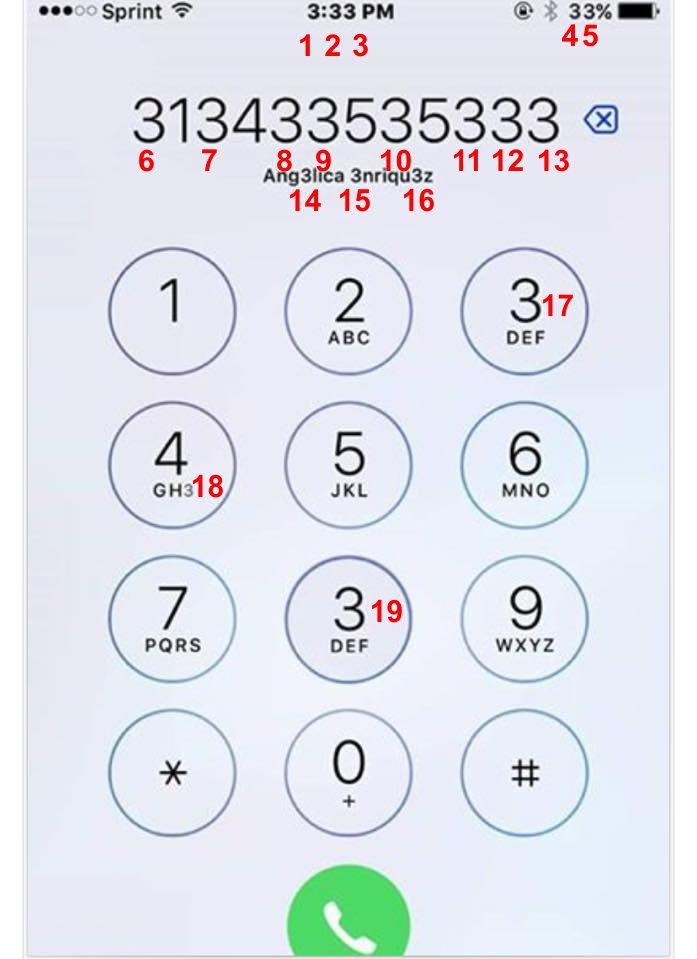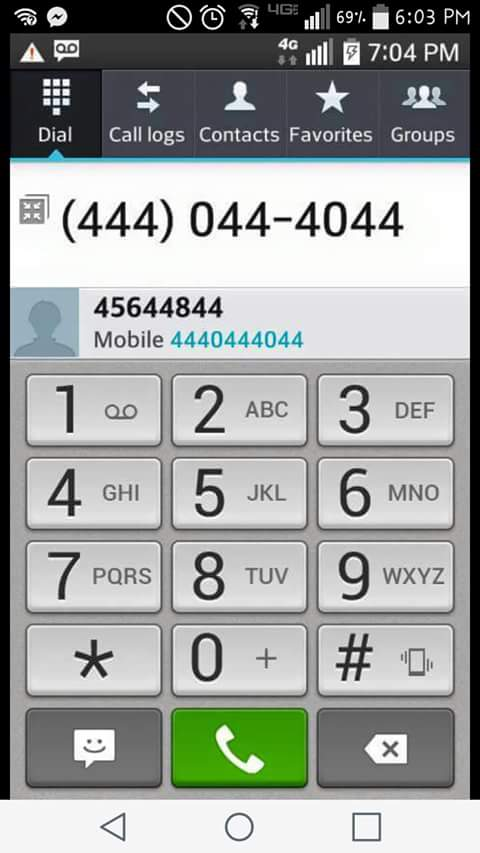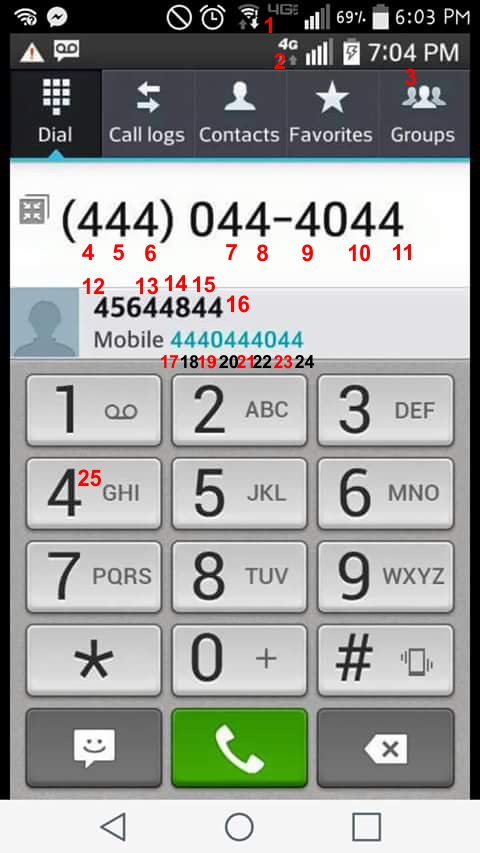# How Many “3” You Can Find in This Picture? (and How many “4”)

Like This:

Test! A graphic shows a smartphone screen and asks us to determine how many 3’s can be seen. First we’ll take a look at the “How Many 3’s” graphic, which has tens of thousands of shares on Facebook as of February 2016.How Many “3” You Can Find in This Picture?

You

can

find

the

answer

bellow.

how many 3’s do you see correct answerHow Many “3” You Can Find in This Picture?

You’ll note that whoever created this graphic sneakily put a “3” in place of the small “I” and in place of the number 8 on the dial pad. I’ve seen some readers claim that the battery’s three dots could be considered a “3” along with the 3 bars on the Sprint signal (because the question doesn’t specifically state to find the number 3, just “3’s”). If you count those, you could make a case for 21 being the answer.

## HOW MANY 4’S?

A similar graphic has also circulated with nearly as many shares as the “count the 3’s” graphic above. This one asks us to count the number 4.How Many “3” You Can Find in This Picture? (and How many “4”)

I found a solution of 25, as shown in the graphic below. It appears that some people miss the faint 4G at the top of the graphic, so you’ll see a number of readers giving an answer of 24.How Many “3” You Can Find in This Picture? (and How many “4”)

Again, if you count signal strength, you could come up with a solution that’s a couple of numbers higher than this.

How Many “3” You Can Find in This Picture? (and How many “4”)
4.8 (95.28%) 127 votes